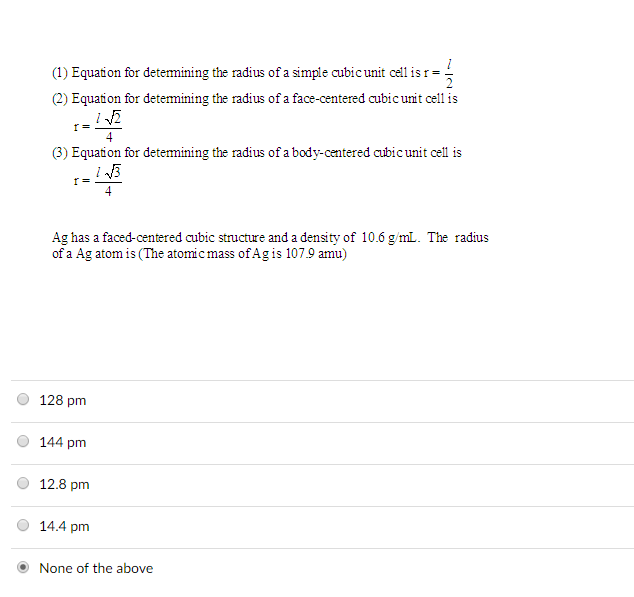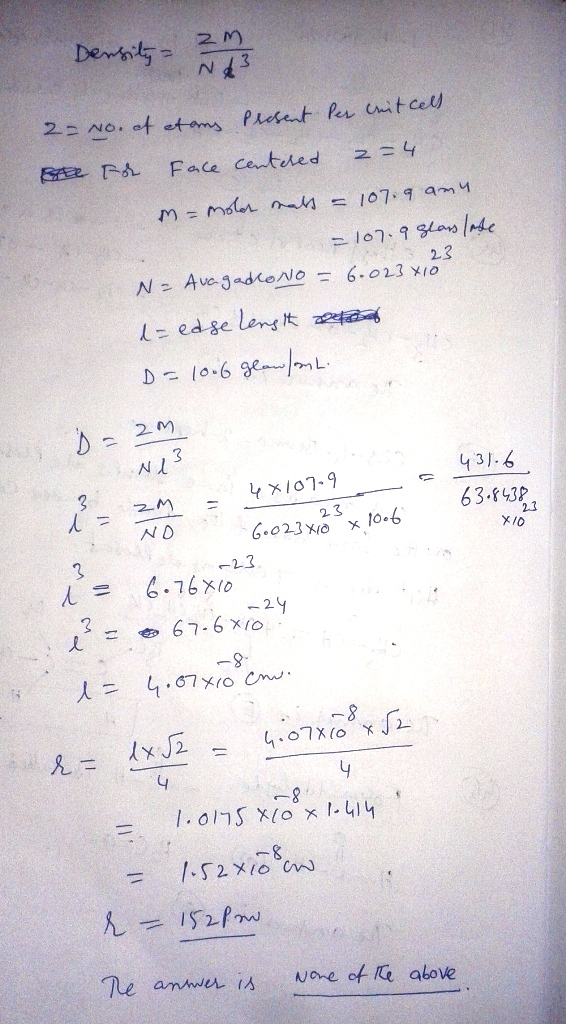# Question & Answer: Equation for determining the radius of a simple cubic unit cell is r = l/2 Equation for…..Equation for determining the radius of a simple cubic unit cell is r = l/2 Equation for determining the radius of a face-centered cubic unit cell is r = l squareroot 2/4 Equation for determining the radius of a body-centered cubic unit cell is r = l squareroot 3/4 Ag has a faced-centered cubic structure and a density of 10.6 g/mL. The radius of a Ag atom is (The atomic mass of Ag is 107.9 amu) 128 pm 144 pm 12.8 pm 14.4 pm None of the above